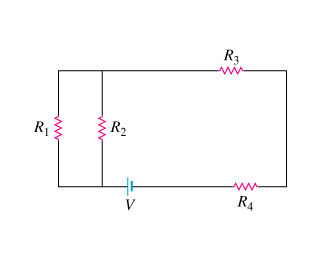# Problem: Consider the network of four resistors shown in the diagram, where R1 = 2.00Ω , R2 = 5.00Ω , R3 = 1.00Ω , and R4 = 7.00Ω . The resistors are connected to a constant voltage of magnitude V. (Figure 1)A. Find the equivalent resistance RA of the resistor network.B. Two resistors of resistance R5 = 3.00Ω and R6 = 3.00Ω are added to the network, and an additional resistor of resistance R7 = 3.00Ω is connected by a switch, as shown in the diagram..(Figure 2) Find the equivalent resistance RB of the new resistor network when the switch is open.C. Find the equivalent resistance RC of the resistor network described in Part B when the switch is closed.

###### FREE Expert Solution

Equivalent resistance for resistors in parallel:

$\overline{)\frac{\mathbf{1}}{{\mathbf{R}}_{\mathbf{eq}}}{\mathbf{=}}\frac{\mathbf{1}}{{\mathbf{R}}_{\mathbf{1}}}{\mathbf{+}}\frac{\mathbf{1}}{{\mathbf{R}}_{\mathbf{2}}}{\mathbf{+}}{\mathbf{.}}{\mathbf{.}}{\mathbf{.}}{\mathbf{+}}\frac{\mathbf{1}}{{\mathbf{R}}_{\mathbf{n}}}}$

or for 2 resistors:

$\overline{){{\mathbf{R}}}_{{\mathbf{eq}}}{\mathbf{=}}\frac{{\mathbf{R}}_{\mathbf{1}}{\mathbf{R}}_{\mathbf{2}}}{{\mathbf{R}}_{\mathbf{1}}\mathbf{+}{\mathbf{R}}_{\mathbf{2}}}}$

Equivalent resistance for resistors in series:

$\overline{){{\mathbf{R}}}_{{\mathbf{eq}}}{\mathbf{=}}{{\mathbf{R}}}_{{\mathbf{1}}}{\mathbf{+}}{{\mathbf{R}}}_{{\mathbf{2}}}{\mathbf{+}}{\mathbf{.}}{\mathbf{.}}{\mathbf{.}}{\mathbf{+}}{{\mathbf{R}}}_{{\mathbf{n}}}}$

A.

R1 and R2 are in parallel.

R12 is in series with R3 and R4

RA = R1R2/(R1 + R2) + R3 + R4

96% (101 ratings)###### Problem DetailsConsider the network of four resistors shown in the diagram, where R1 = 2.00Ω , R2 = 5.00Ω , R3 = 1.00Ω , and R4 = 7.00Ω . The resistors are connected to a constant voltage of magnitude V. (Figure 1)

A. Find the equivalent resistance RA of the resistor network.B. Two resistors of resistance R5 = 3.00Ω and R6 = 3.00Ω are added to the network, and an additional resistor of resistance R7 = 3.00Ω is connected by a switch, as shown in the diagram..(Figure 2) Find the equivalent resistance RB of the new resistor network when the switch is open.

C. Find the equivalent resistance RC of the resistor network described in Part B when the switch is closed.